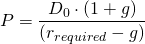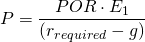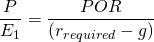# PE ratio

The PE ratio, which is shorthand for price earnings ratio, indicates how expensive or cheap a certain security is. The PE ratio can be calculated by dividing the price per share obtained from the exchange by the earnings per share. This ratio measures how many times the current earnings investors are prepared to pay.

An investment in shares with low a PE ratio is expected to earn its investment back  faster than an investment in shares with a high PE ratio. Usually shares with a PE ratio below 10 are categorized as cheap, while those with a PE ratio higher than 20 are considered to be expensive. Of course, when analysing the price earnings ratio, this should be done relative to the sector average in which the company is active.

Additionally, PE ratios calculated above are lagging indicators. They rely upon earnings reported in the previous year. Investors thus need to either form an expectation on the company’s future earnings, or extract this from the market using a valuation model such as a dividend discount model.

## Forward-looking PE

Using the dividend discount model, we can determine the intrinsic value of a share (P) using the last paid dividend (D0), the required rate of return (rrequired) and optionally the growth rate in dividends (g). Recall that the next years dividend D1 equals the current dividend adjusted for growth.As mentioned before, the future dividend in itself is just a fraction of the future earning (E1). The dividend can be determined by multiplying the expected earning by the company’s payout ratio, POR.From this follows that the forward-looking PE ratio equals the payout ratio divided by the required return accommodated from dividend growth.## Summary

The PE ratio is a lagging indicator often used in the financial markets which measures how expensive a certain share is.

### PE ratio

Need to have more insights? Download our free excel file: PE ratio.